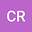•A generalized Friedmann-Lemaître-Robertson-Walker metric specifies gravitational fields with equations of state of w = −1. In the spacetime of the perturbed Robertson-Walker metric, the gravitational pressure is negative. A gravitational positive feedback cycle, recognized in Einstein’s seminal work – The Foundation of the Theory of General Relativity - gives rise to the self-induction of gravity that, under conservation of gravitational energy density, is proposed to lead to continuous growth of the field, even in the absence of changes in gravitating bodies. Physical space is proposed to be the form of the field. This auto-induced growth of the field is, here, proposed to manifest as the expansion of space – elsewhere attributed to dark energy. A gravitational expansion, by virtue of the conservation of gravitational energy density and the equation of state, is locally isobaric. The Hubble-Lemaître law is derived from the isobaric nature of the expansion. Data from cosmological probes across z = 0 to z > 9 support the linear redshift-distance relation that demands a cosmic Hubble constant. The Hubble constant references a universal maximum gravitational energy density ~ 1.52E-27 Kg m-3 @ H2.26E-18 s-1. It is proposed that geodesics are dependent on the conserved gravitational energy density. Thereby, the expansion of the field in the vicinities of bodies leads to extended flat, or rising, orbital velocity profiles and extensive regions of gravitational lensing – elsewhere attributed to dark matter. A gravitational expansion would impart a historical character to the laws of gravitation. In this spacetime, continuous gravitational expansion is essential in preventing spatial collapse.